# Calculation methods

ELPLA can be used to analyze raft/piled raft or any other structural problems such as slab floors, grids, plane frame, plane stress, a system of many slab foundations, rotational shell, axisymmetric stress, and axisymmetric structures (Figure 2)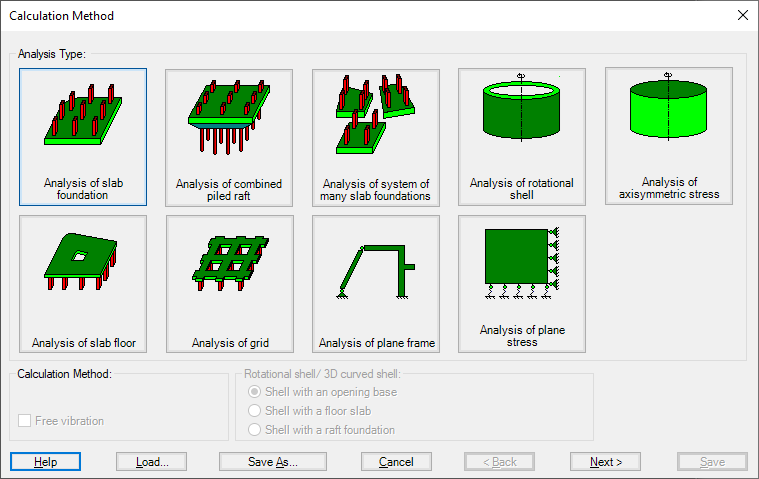Figure 2 Calculation Methods
The analysis of slab foundation problems is available in ELPLA (Figure 3 and Figure 4).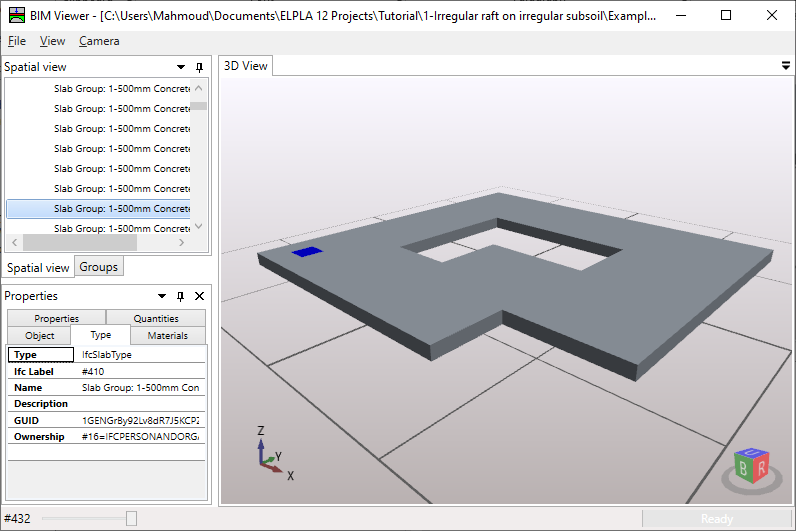Figure 3 "Analysis of slab foundation"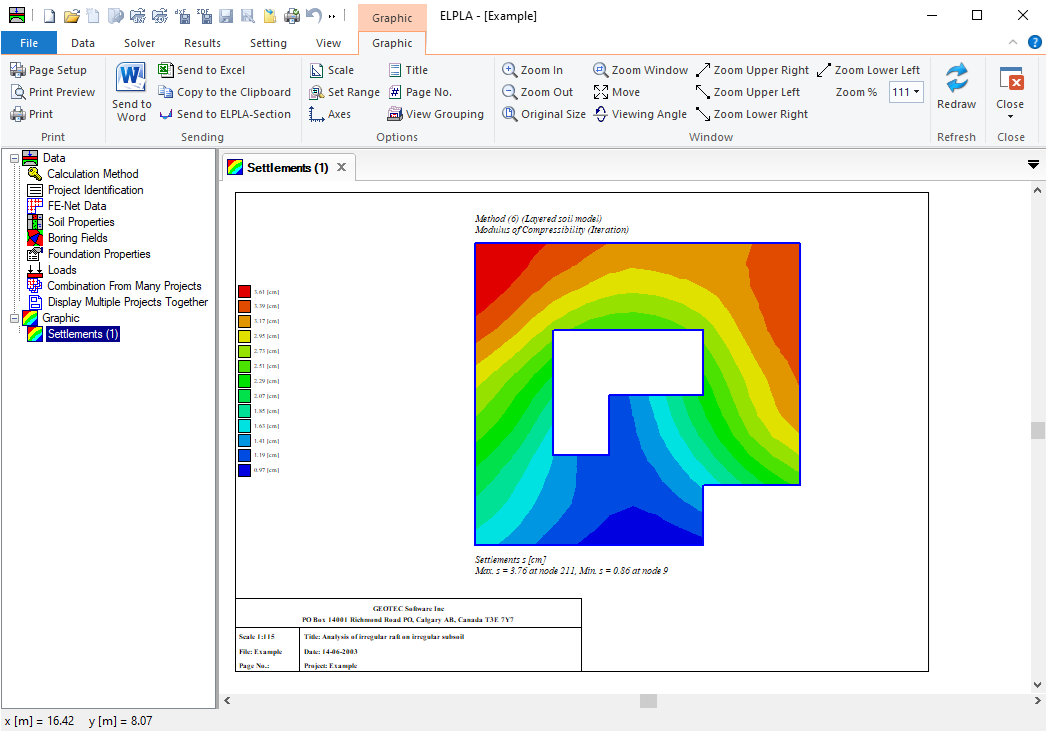Figure 4 "Analysis of slab foundation" displacements
The analysis of slab floor problems is available in ELPLA (Figure 5 and Figure 6).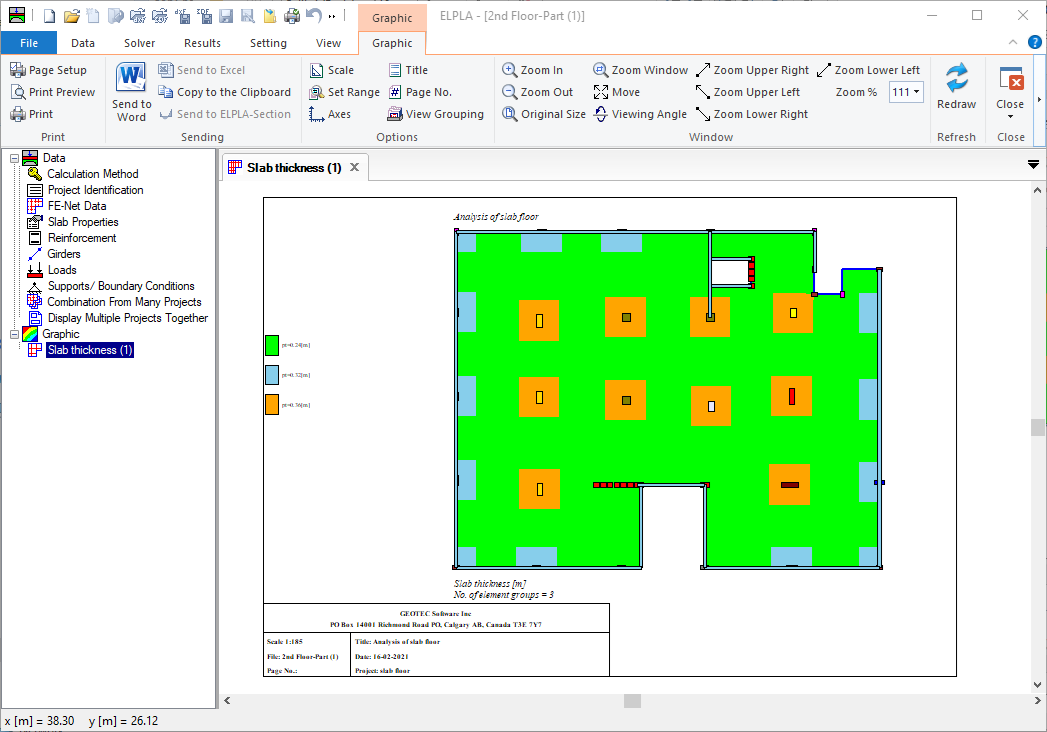Figure 5 "Analysis of slab floor" slab thickness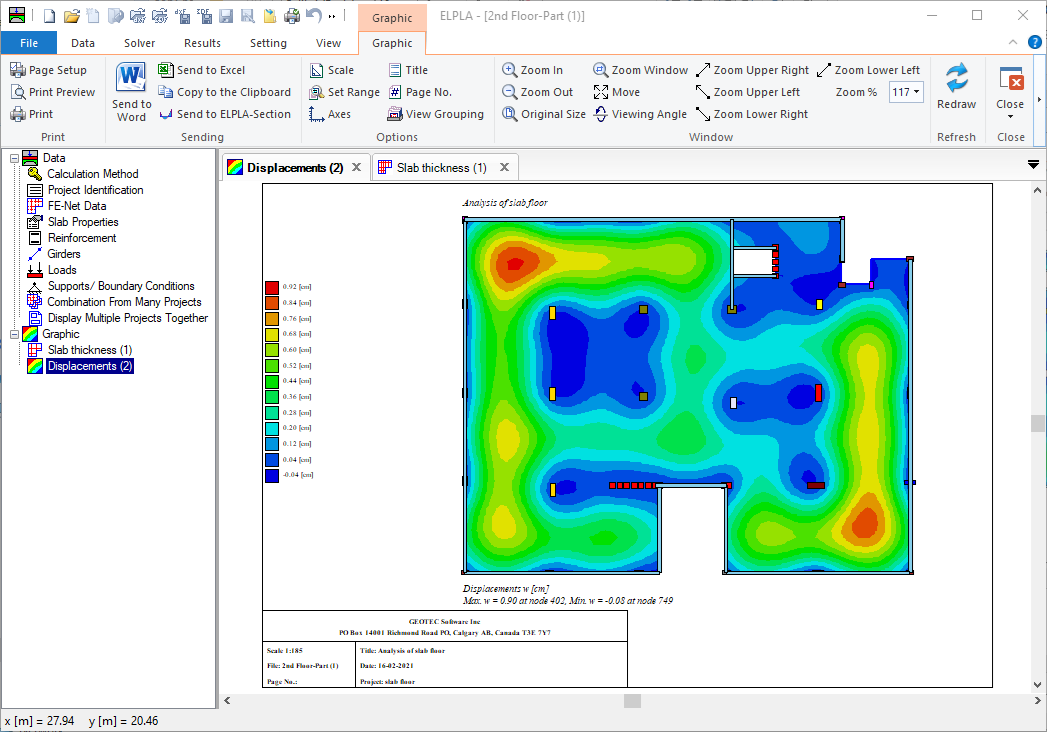Figure 6 "Analysis of slab floor" displacements
The analysis of piled raft problems is available in ELPLA (Figure 7 and Figure 8).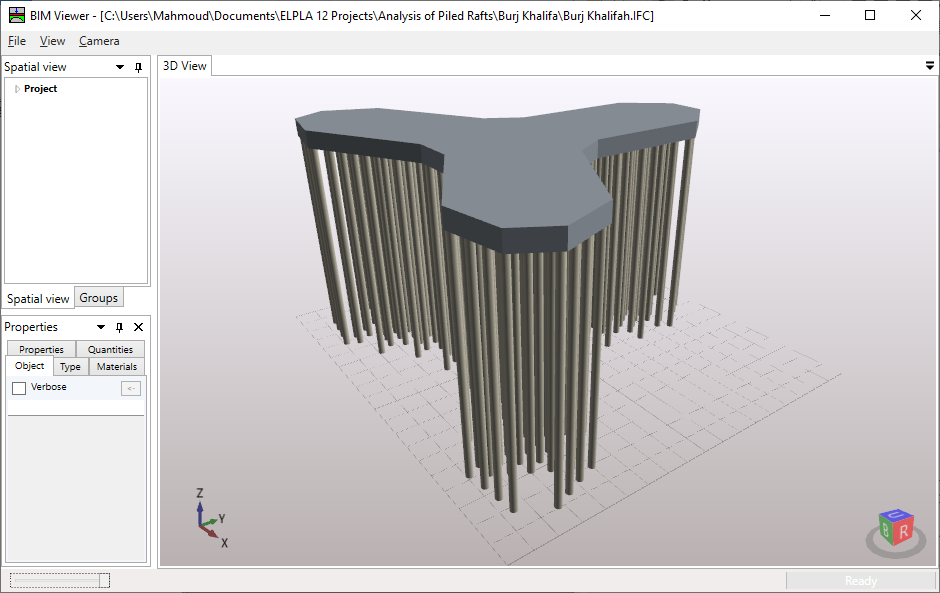Figure 7 "Analysis of Burj Khalifah foundation"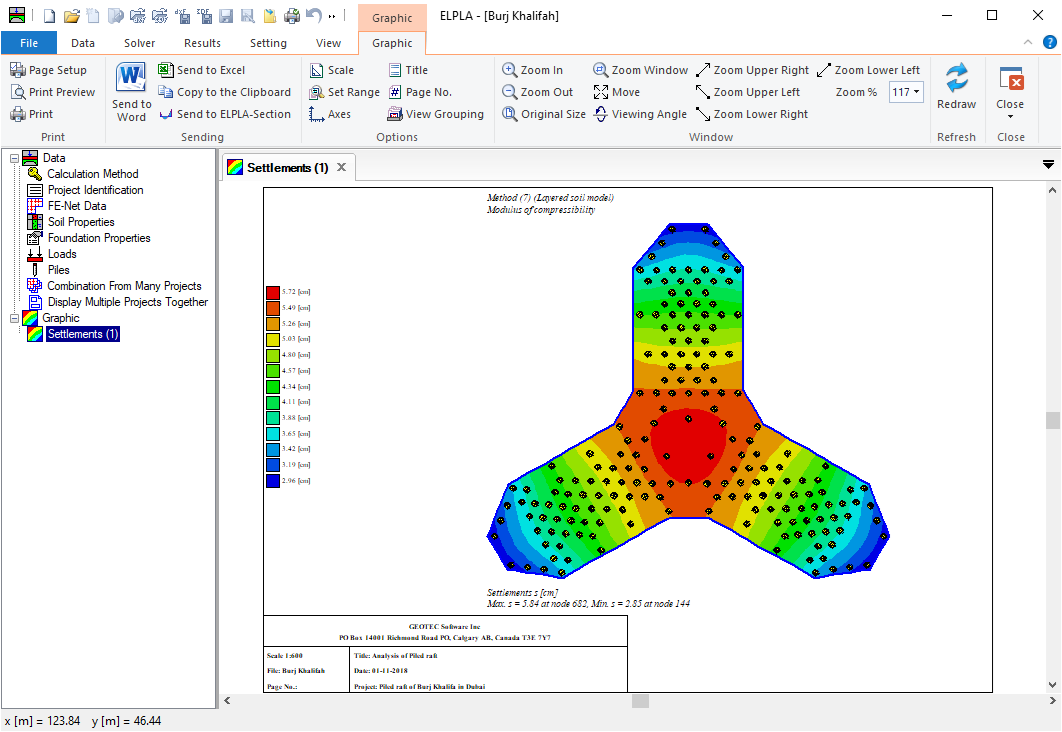Figure 8 "Analysis of Burj Khalifah foundation" displacements
The analysis of grid problems is available in ELPLA (Figure 9).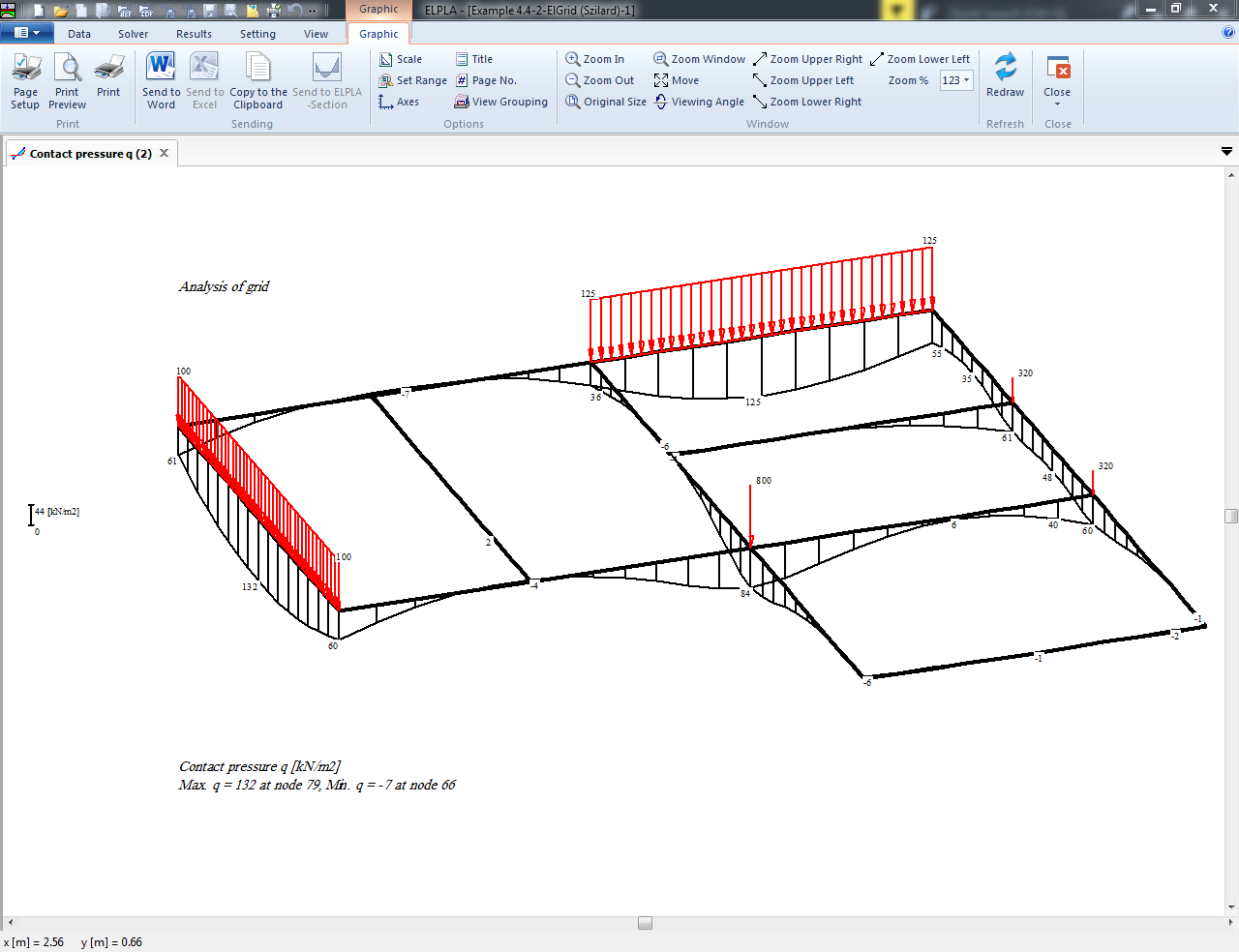Figure 9 "Analysis of grid"
In the "Analysis type" Form, if the option "Analysis of system of many slab foundations" is chosen, the following Dialog box in Figure 10 appears. Three different numerical calculation methods are considered for the analysis of a system of slab foundations, flexible, elastic, or rigid. For the analysis of a system of many slab foundations, the project filenames (slab foundations) are required. Figure 11.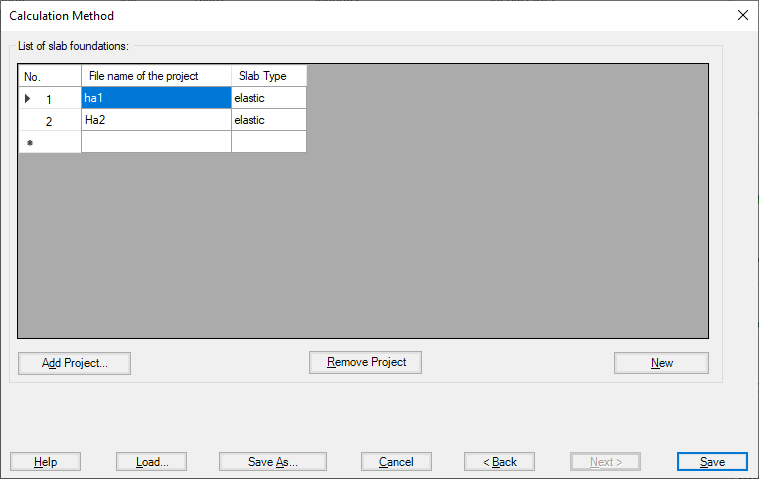Figure 10 "Analysis of system of many slab foundations" Dialog box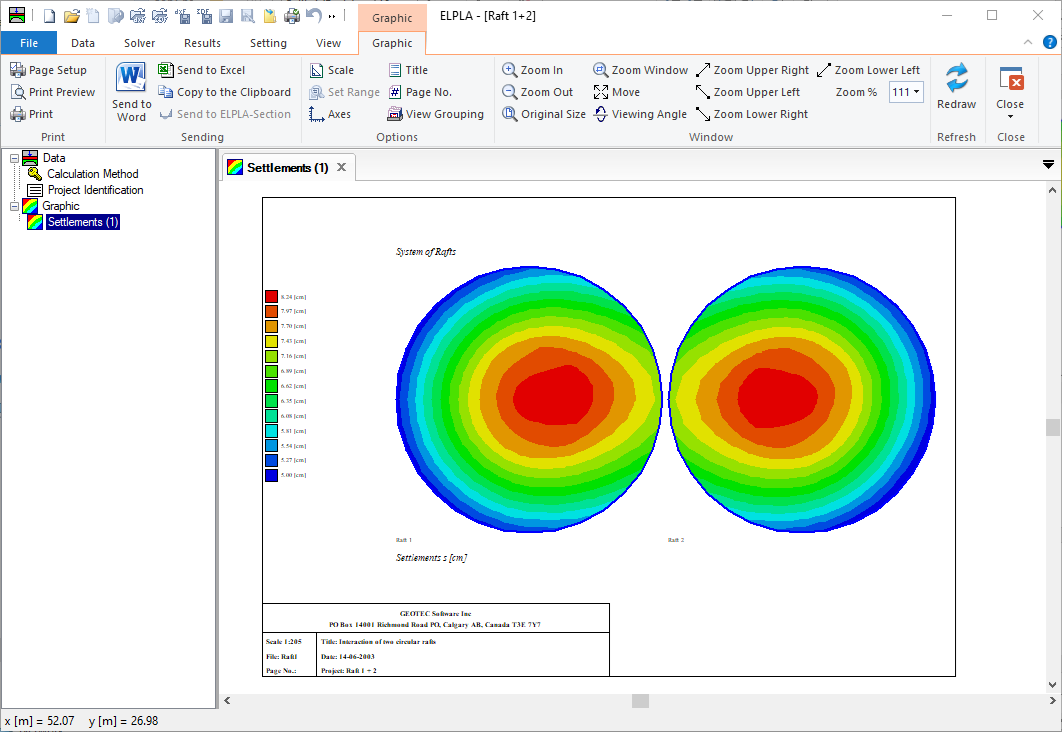Figure 11 "Analysis of system of many slab foundations" displacements
The analysis of plane stress problems is available in ELPLA (Figure 12).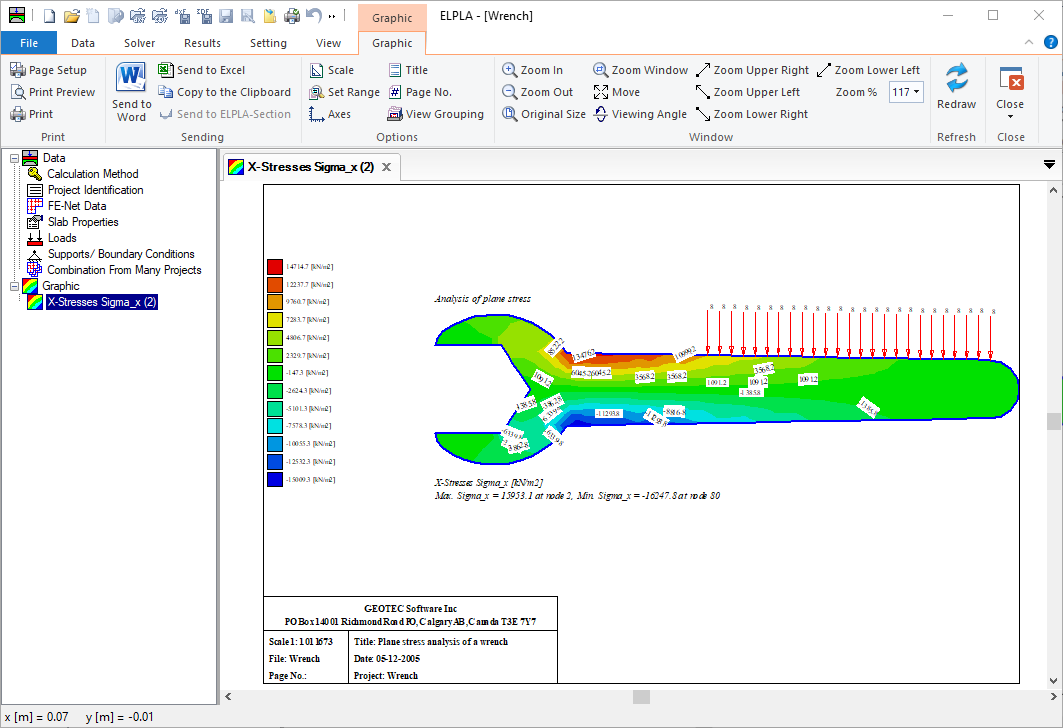Figure 12 Analysis of plane stress
The analysis of Two-Dimensional frame problems is available in ELPLA (Figure 13).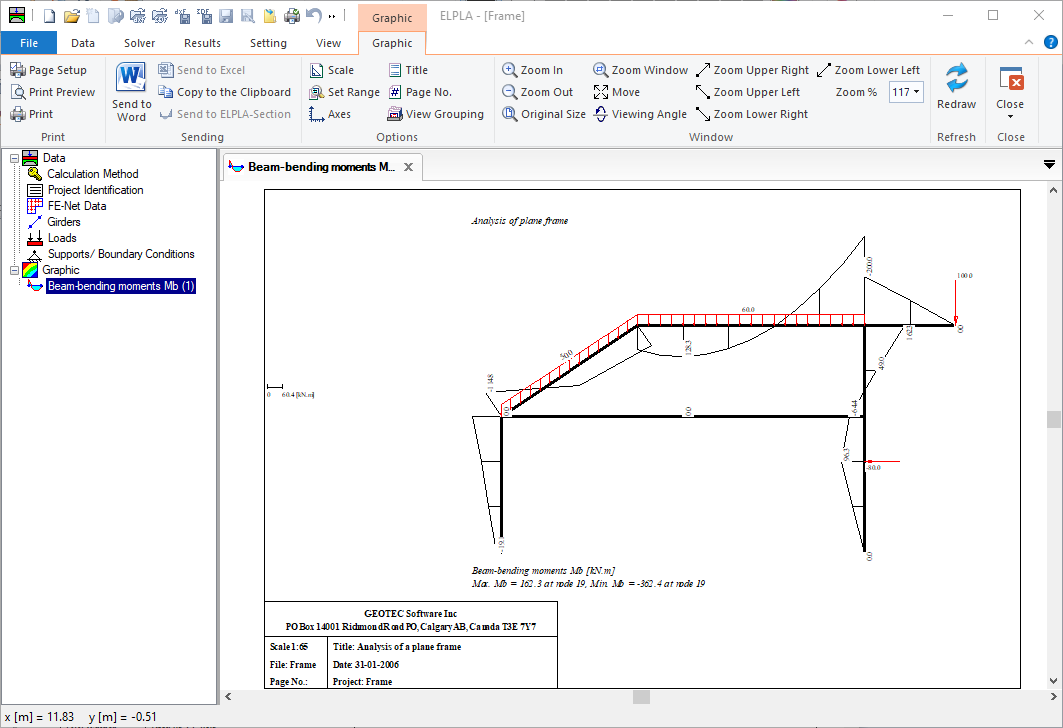Figure 13 Analysis of plane frame
It is possible to determine Eigenmodes and Eigenvectors due to free vibration for the following structures:
1. Beams
2. Trusses
3. Grids
4. Space frames
5. Shear walls
6. Floor slabs
7. Axisymmetric solids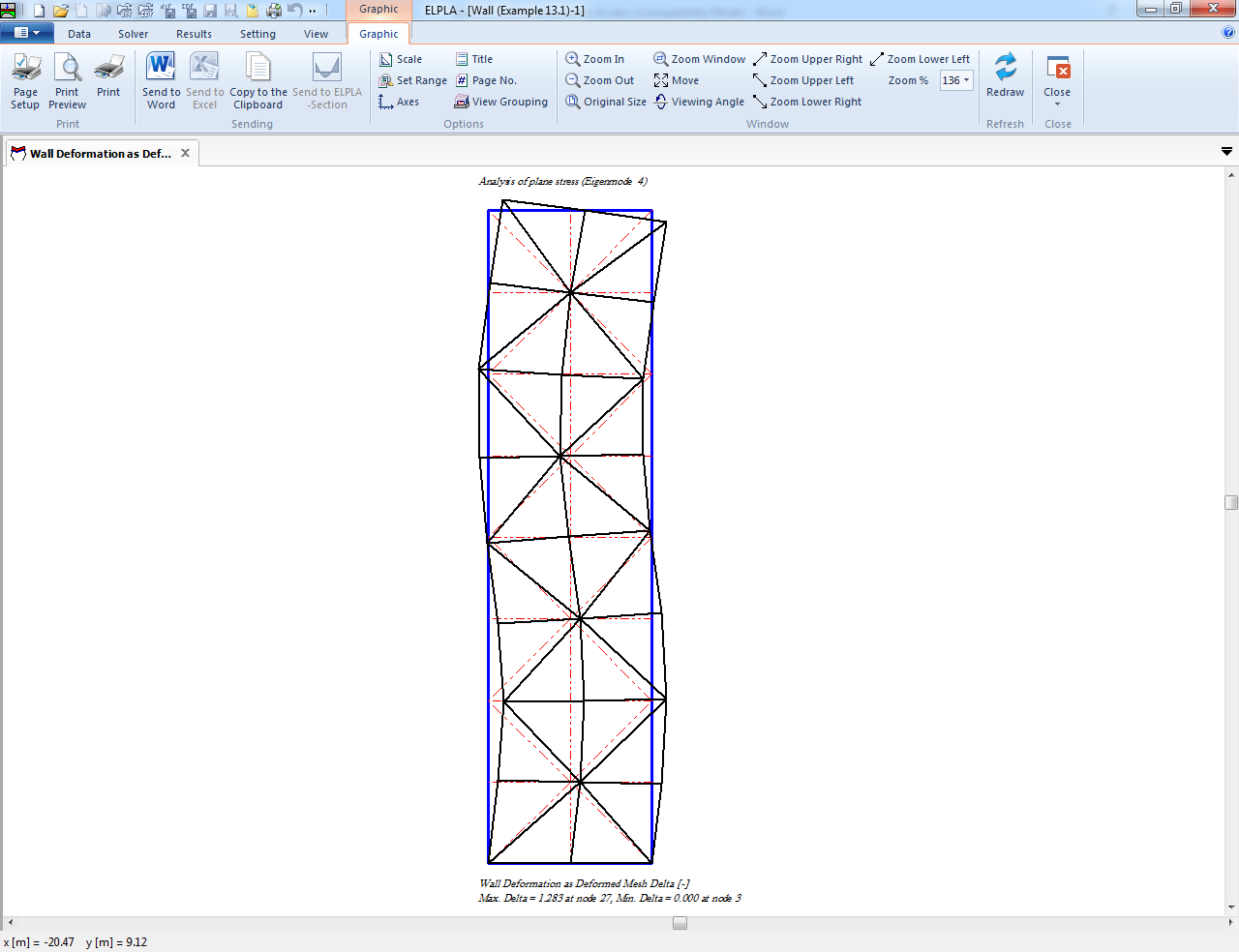Figure 14 Dynamic analysis of shear wall
The next step is to define the "System symmetry", Figure 15. In this step, select system symmetry and click "Next" button to go to the next step.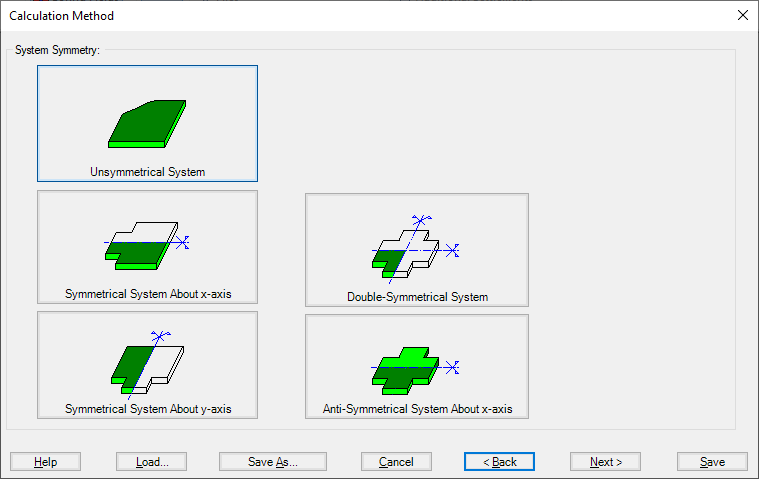Figure 15 "System Symmetry" Form
By using the system symmetry, if the problem is symmetrical in loading, shape, and soil about x- or y-axis, the computational time and computer storage can be considerably reduced.
By defining the project data for a simple symmetrical or anti-symmetric slab system, the data are defined according to Figure 16, in which only the lower half slab is considered for symmetry about the x-axis while only the left half slab is considered for symmetry about the y-axis.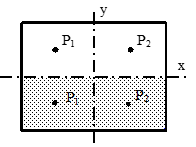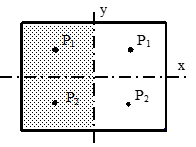Figure 16 Simple symmetrical slab system

By defining the project data for a double symmetrical slab system, the data are defined according to Figure 17. Only the left lower quarter of the slab is considered.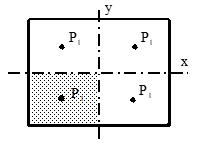Figure 17 Double symmetrical loaded slab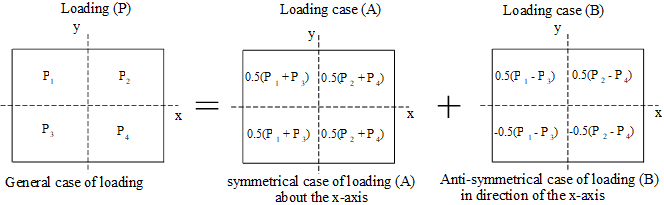The symmetrical cases are available for all calculation methods 1 to 9. The anti-symmetric case is only possible for calculation methods 4 to 8.
Some options are available in ELPLA such as the concrete design of sections, additional springs, supports, girders, piles, limit depth, nonlinear subsoil model, determining displacements, stresses, and strains in soil. Also, ELPLA can study some external influences on the raft such as temperature change, additional settlements, or neighboring foundations. In the menu of Figure 19 check the options that you want to consider in the analysis.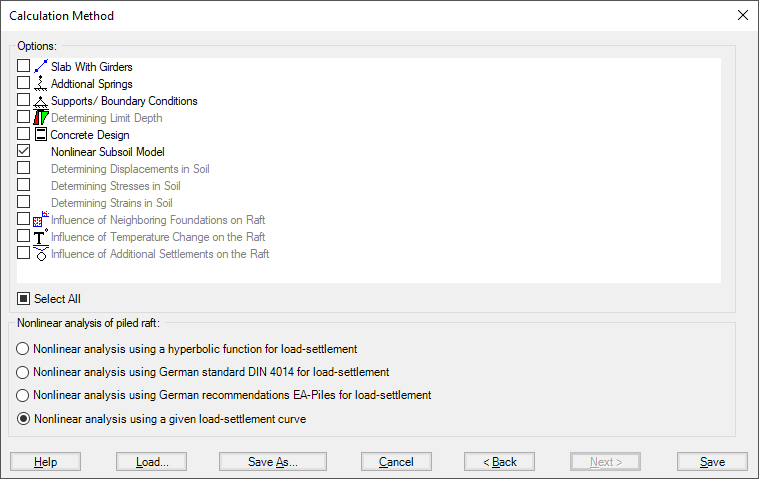Figure 19 "Options" Check box